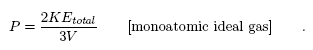Lectures on Physics has been derived from Benjamin Crowell's Light and Matter series of free introductory textbooks on physics. See the editorial for more information....# Pressure, volume, and temperature

A gas exerts pressure on the walls of its container, and in the kinetic theory we interpret this apparently constant pressure as the averaged-out result of vast numbers of collisions occurring every second between the gas molecules and the walls. The empirical facts about gases can be summarized by the relation

PV α nT, [ideal gas]

which really only holds exactly for an ideal gas. Here n is the number of molecules in the sample of gas.Volume related to temperature

We now connect these empirical facts to the kinetic theory of a classical ideal gas. For simplicity, we assume that the gas is monoatomic (i.e., each molecule has only one atom), and that it is confined to a cubical box of volume V , with L being the length of each edge and A the area of any wall. An atom whose velocity has an x component vx will collide regularly with the left-hand wall, traveling a distance 2L parallel to the x axis between collisions with that wall. The time between collisions is Δt = 2L/vx , and in each collision the x component of the atom's momentum is reversed from -mvx to mvx. The total force on the wall iswhere the indices 1, 2, . . . refer to the individual atoms. Substituting Δpx,i = 2mvx,i and Δti = 2L/vx,i, we haveThe quantity mv2x,i is twice the contribution to the kinetic energy from the part of the atom's center of mass motion that is parallel to the x axis. Since we're assuming a monoatomic gas, center of mass motion is the only type of motion that gives rise to kinetic energy. (A more complex molecule could rotate and vibrate as well.) If the quantity in parentheses included the y and z components, it would be twice the total kinetic energy of all the molecules. By symmetry, it must therefore equal 2/3 of the total kinetic energy, soDividing by A and using AL = V , we haveThis can be connected to the empirical relation PV α nT if we multiply by V on both sides and rewrite KEtotal as nKEav, where KEav is the average kinetic energy per molecule:For the first time we have an interpretation for the temperature based on a microscopic description of matter: in a monoatomic ideal gas, the temperature is a measure of the average kinetic energy per molecule. The proportionality between the two is KEav = (3/2)kT, where the constant of proportionality k, known as Boltzmann's constant, has a numerical value of 1.38×10-23 J/K. In terms of Boltzmann's constant, the relationship among the bulk quantities for an ideal gas becomes

PV = nkT , [ideal gas]

which is known as the ideal gas law. Although I won't prove it here, this equation applies to all ideal gases, even though the derivation assumed a monoatomic ideal gas in a cubical box. (You may have seen it written elsewhere as PV = NRT, where N = n/NA is the number of moles of atoms, R = kNA, and NA = 6.0 × 1023, called Avogadro's number, is essentially the number of hydrogen atoms in 1 g of hydrogen.)Pressure in a car tireEarth's senescenceA piston, a refrigerator, and a space suit

Last Update: 2011-01-31